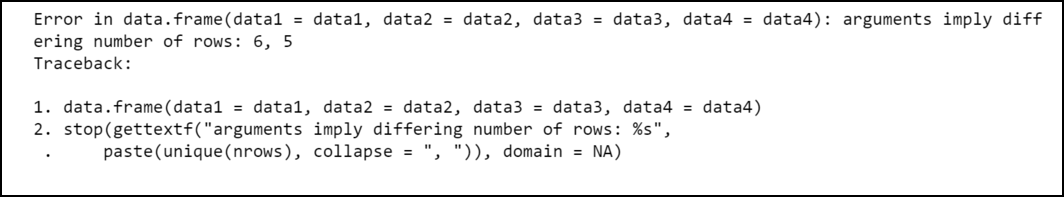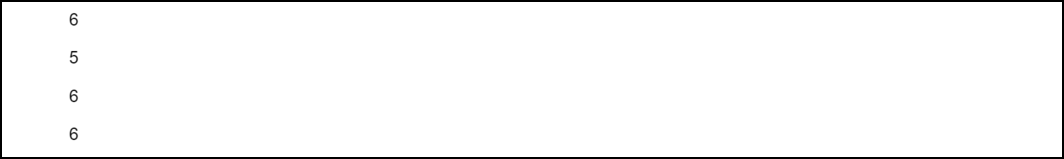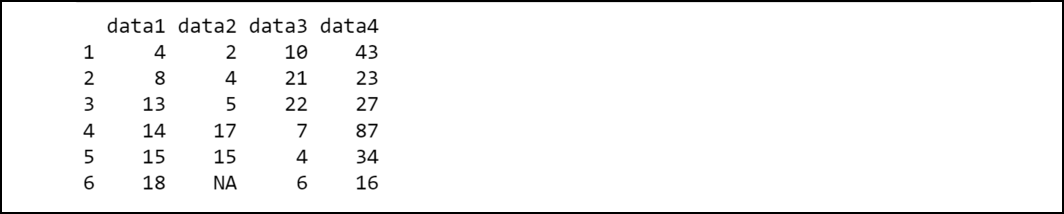GFG App
Open AppBrowser
Continue

# How to Fix in R: Arguments imply differing number of rows

In this article, we are going to see how to fix  Arguments that imply differing numbers of rows in R Programming Language.

One error that we may encounter in R is:

arguments imply differing number of rows: 6, 5

The compiler produces such an error when we try to create a data frame and the number of rows in each column of the data frame differs from each other.

## When this error might occur

Let’s try to create a data frame in R using four-vectors.

## R

 `# Define vectors ` `data1 <- ``c``(4, 8, 13, 14, 15, 18) ` `data2 <- ``c``(2, 4, 5, 17, 15) ` `data3 <- ``c``(10, 21, 22, 7, 4, 6) ` `data4 <- ``c``(43, 23, 27, 87, 34, 16) ` ` `  `# Try to create data frame with vectors  ` `# as columns ` `df <- ``data.frame``(data1=data1, data2=data2, ` `                 ``data3=data3, data4=data4) `

Output:The compiler produces such an error because the length of the vectors used is not the same. Hence, the numbers of rows in the columns are not the same. We can also cross-check this by printing the length of the vectors.

## R

 `# Define vectors ` `data1 <- ``c``(4, 8, 13, 14, 15, 18) ` `data2 <- ``c``(2, 4, 5, 17, 15) ` `data3 <- ``c``(10, 21, 22, 7, 4, 6) ` `data4 <- ``c``(43, 23, 27, 87, 34, 16) ` ` `  `# Print the length ` `length``(data1) ` `length``(data2) ` `length``(data3) ` `length``(data4) `

Output:As we can see in the output data1, data3, data4 has a length equal to 6 but data2 has a length equal to 5.

## How to Fix the Error?

This error can be fixed easily by keeping in mind that each vector must have the sample length so that each column in the final data frame would have the number of rows. For example, we could pad the shortest vector with NA values so that each vector has the same length:

## R

 `# Define vectors ` `data1 <- ``c``(4, 8, 13, 14, 15, 18) ` `data2 <- ``c``(2, 4, 5, 17, 15) ` `data3 <- ``c``(10, 21, 22, 7, 4, 6) ` `data4 <- ``c``(43, 23, 27, 87, 34, 16) ` ` `  `# Configure the shortest vector with NA's ` `# to have same length as longest vector ` `length``(data2) <- ``length``(data1) ` ` `  `# Try to create data frame with vectors ` `# as columns ` `df <- ``data.frame``(data1=data1, data2=data2,  ` `                 ``data3=data3, data4=data4) `

Output:The program compiled successfully this time as each column has the same number of rows in the data frame.

My Personal Notes arrow_drop_up